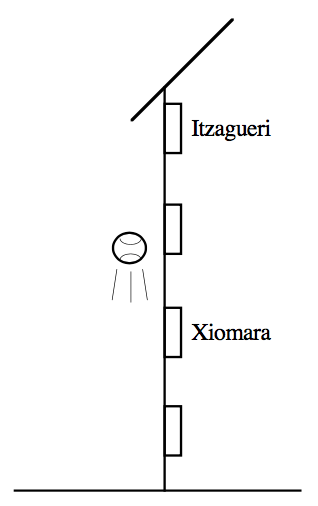### Home > APCALC > Chapter 6 > Lesson 6.2.2 > Problem6-74

6-74.

From her second floor window $6$ meters above the ground, Xiomara throws a tennis ball at a rate of $11$ meters per second up towards her friend Itzagueri who is $12$ meters above the ground. Assume $a(t) = -9.8$ meters per $\sec^2$ for the acceleration due to gravity.

1. Assuming Itzagueri does not catch the ball on its way up, describe the motion of the ball $2$ seconds after it was thrown.

At $t = 2$, is the ball rising or falling? Is its velocity increasing or decreasing?

2. When does the ball reach its highest point?

The ball stops moving at its highest point.

The height is given by $h(t) = -4.9t^2 + 11t + 6$.
h(answer from part (b)) $≈ 12.173$ m; so yes.

4. Determine the height of the ball is when it is falling at the rate of $5$ meters per second.

Before you evaluate the height, find the time when
$v(t) = -5$.

5. Explain what $\int _ { 0 } ^ { 2 } a ( t ) d t$ represents physically.

Be sure to explain the significance of BOTH the integrand and the bounds.